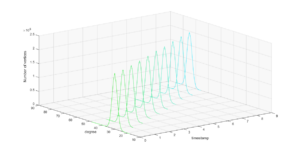# Datasets

Dataset 1:

In this dataset, we control the degree distribution of a sequence of 10 successive snapshots with the following parameters:

Gaussian distribution parameters:
N_0 = 1M
Mu_0 = 30
Sigma_0 = 5

deltaN = 0
deltaMu = 3
demtaSigma = 0

Htree parameters:
b = 2
h = 2
k = 20
c = 4

Note that these parameters translates to a sequence of growth only snapshots where the average degree increases by 3 between each pair of successive snapshots.

The figure bellow shows the evolution of the degree distribution of the generated graph snapshots.